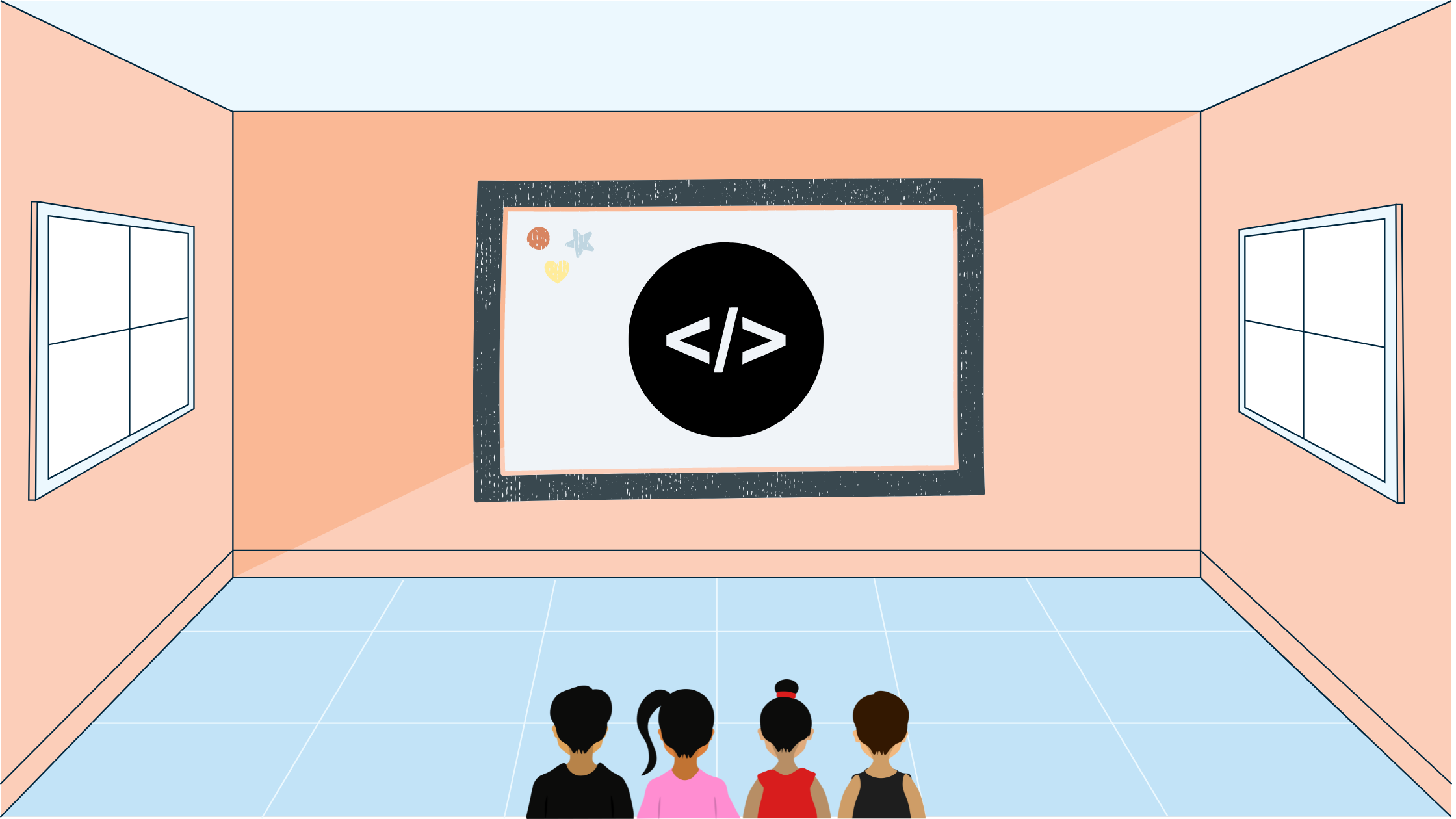Yash Singh# USACO Alchemy

In this blog post I'll go over the USACO Alchemy Bronze US Open 2022 Problem 3 using other resources and solutions.

## Statement

A cow has some amount of several metals, each metal numbered from 1 through $N$. A metal with a higher number has a higher value. The cow also knows several recipes for converting some one or more metals into a metal that is of more value than the ingredients. Our goal is to return the maximum number of metal $N$ the cow can get by applying transformations through the given recipe.

## Solution

Let's visualize the recipe structure as a graph for the following recipes.

5 = 3 + 4
3 = 1 + 2
4 = 1We cannot iterate bottom-up in this graph because we will not know where to start from, so we will have to start from the top. We have to do a depth-first search of this graph because each node depends on its children (if they exist).

Let's go through an example depth-first search for crafting 5. We start at 5, and move on to 4.Next, we move to 1 and convert all of the 1 nodes into 4 nodes. Now, we have zero nodes with ID 1.Now we move up and back to 3. However, 3 cannot be crafted because to make one metal 3 you need one metal 1 and one metal 2, however we used up all of our metal 1's to craft metal 4's. Handling all the dependency logic can become pretty complicated and we can end up in tons of edge cases.

If we look at the maximum units of metal the cow has for each metal type it's $10^4$. What if we did a depth-first search (which takes $O(N^2)$ which is $10^4$ operations) for each unit of metal we want to decrement? That will take us only $10^4\times10^4=10^8$ operations. That will be underneath the $2\times10^8$ operations limit that USACO gives us for C++. We can recursively go down the graph and increment the metal count by 1 if the ingredients are avaliable.

## Implementation

Let's go through an implementation in C++:

#include <bits/stdc++.h>

using namespace std;

// q: quantities, r: recipes
int q;
vector<int> r;

bool cook(int pos) {
// If this is a leaf node that can't be created
// we have to rely on the given supply in that case
if (r[pos].empty()) {
if (q[pos] == 0) {
return false;
}
--q[pos];
return true;
}
// If we have existing supply
if (q[pos] != 0) {
--q[pos];
return true;
}
// No existing supply, but yes recipe
for (auto ing: r[pos]) {
// Does the ingredient have an existing supply
if (q[ing] > 0) {
--q[ing];
}
// Otherwise try to cook the ingredient
else if (!cook(ing)) {
return false;
}
}
return true;
}

int main() {
// Read in quantities, recipes, and other input
ios_base::sync_with_stdio(false);
cin.tie(nullptr);
int n;
cin >> n;

for (int i{0}; i < n; ++i) {
cin >> q[i + 1];
}

int k;
cin >> k;
while (k--) {
vector<int> re;
int to, qua;
cin >> to >> qua;
while (qua--) {
int x;
cin >> x;
re.push_back(x);
}
r[to] = re;
}

// Keep cooking metal N until it isn't possible anymore
// In that case the function cook will return false
int ans{0};
while (cook(n)) {
++ans;
}

cout << ans;

return 0;
}

## Lessons Learnt

A lesson learned in this problem is to try and visualize the input. Also, check each and every possible naive method to see if it might be under the given time limit.